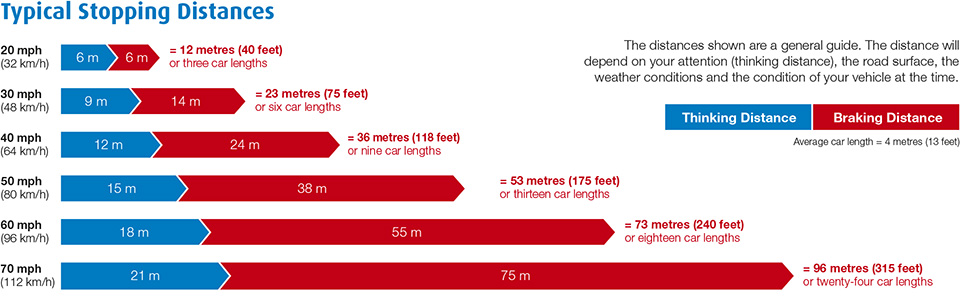# Reblog: Know Your Range aka How to Set Your Stop Loss ⋆ 5-To-9 TraderThere is nothing more frustrating than getting stopped out of a trade only to watch the price go back to the initial direction you were trading in the first place.It’s grating, I know.

Assets, like animals, have different types of characteristics. Some are fast, some are slow, some jump high, some jump low. Understanding the behaviours and patterns of your chosen assets will help you set your stops at reasonable levels.

With this post, I’ll share a simple method of placing your stops in a way that is realistic and decreases the chances of getting hit every single time.

The Concept of Stopping Distances

If you are driving at 50 km/h (~31 mph), do you know how much safety distance you have to maintain between you and the vehicle in front of you ?

What about when you’re cruising at 120kmh (~75mph), will you still be keeping the same distance?

Well, duh!” I hear you say.

The typical stopping distances chart below is a case in point:Your stopping distance should be adjusted to your vehicle’s size and driving speed. For example, larger vehicles weighing 40 tonnes need a greater stopping distance than a compact car that is significantly smaller in weight and size.

A similar concept can be applied in trading.

Basing your stop loss solely on a hard and fixed number (e.g. x number of pips / x number of points) is like using the same stopping distance FOR EVERY SINGLE VEHICLE. There is a smarter way to calculate your stop loss and the first step is to know the range of your asset.

This is akin to knowing the size of the vehicle you’re driving – it makes it easier for you to get a good idea of what a reasonable stopping distance would be. Knowing the trading range of your asset, or the “average true range” (ATR) as we call it, also helps you select a sensible stop level which can decrease the chances of your trade being stopped out, provided that your bias was set in the right direction.

How to Calculate the Average True Range

So here is a simple step-by-step guide on how to calculate the average true range of any asset (be it stocks, commodities, currencies, etc)

1. Get the historical data for your asset. You will need the data of the open, high, and low numbers .This data set usually always comes as a package called open, high, low, and close (OHLC). You can download these numbers from the following free resources:
2. Subtract the low price of the day from the high price of the same day. The result you get is what you call a “True Range”. This tells you how much the asset moved in a day.3. To get the average true range (ATR) for a certain period, you just repeat step number 2 for the number of days you want to calculate the range for. Then you get the average number of the true ranges within your specified period.  Below is an example for a 20-day ATR, which is equivalent to a whole trading month:Calculating the average true range of an asset tells you how much the asset has moved within a particular period

4. You can then calculate the daily ATR in form of a percentage as follows:a. You add the true range of the latest closed trading day and the trading day priorb. You divide the result by 2c. Then you divide the number by the open level of the latest trading dayThe result you get tells you how much the asset is expected to move on an average day. For example, if your calculated ATR is 1.37 %, that means that the asset is expected to move by 1.37% from the opening price. This is how it would look like on a spreadsheet format:Calculating the average true range of an asset expressed in percentage

5. Once you have the percentage worked out for the different time frames you need (e.g. 3 days, 10 days, 20 days, 1 year, 10 years etc), you can use this % as a stop loss level.In the above example, if we are entering a trade say, at \$985 (the numbers on the spreadsheet are expressed in cents), and we have calculated a 20-day ATR of 2.15 %, then we can set our stop level at \$964 (2.15% of \$985 is \$21, so we subtract \$21 from our entry level to calculate the stop level based on the ATR).

You can apply this technique in many different variations. For example, you can use a 3x ATR as a stop level if you know that your asset is a “wild” one, i.e. extremely volatile. Or you can round up the calculated ATR to the nearest 5 for good measure. Or you can even use the ATR to target a profit level.

Voilá. Now you know how to calculate your “stopping distance”.

Will this guarantee that your stop levels won’t get hit?There are no guarantees for anything and there is certainly no one way of setting the right stop level and nailing it every single time. But I would think of it like this:

Most trades almost always start off with a loss, so when you enter a trade, what you’re essentially doing is you give your position some space to prove itself. This “space” is predetermined by the stop loss you set. Once your position exceeds this “space”, that’s when you know you need to cut it as you will be damaging your trading account or portfolio otherwise.

Giving your trades the appropriate amount of “space” is key as you will otherwise be depriving it of the chance to prove itself. This is where knowing how to calculate your asset’s average true range can help you.

And remember to always trade responsibly.

The original post appears on 5to9trader.com and is available here.